Gradient is an Intertechnik brand. Remarkable are the ribbons, which are really not expensive.

Tweeter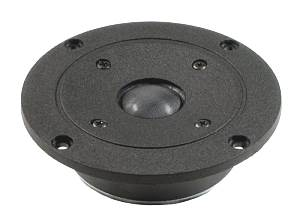GDT 104 F       order no. I-1381611       - not available (_)
25 mm dome tweeter with fabric dome. The voice coil windings are immersed in magnetic fluid to increase short term power handling capacity, to reduce the compression and the Q factor..
• power handling (continuous/programme) = 45 W
• frequency range = 2500-18000 Hz
• impedance R = 8 Ohm
• sound pressure level SPL = 91 dB (2,83V; 1m)
• voice coil diameter = 25 mm
• mounting diameter d = 81 mm
• overall diameter d = 104 mm
• mounting depth (not countersunk) t = 32 mm
PDF data sheetGDT 104 F/TV       order no. I-1381621       - not available (_)
25 mm dome tweeter with fabric dome. The voice coil windings are immersed in magnetic fluid to increase short term power handling capacity, to reduce the compression and the Q factor.. The driver is magnetically shielded.
• power handling (continuous/programme) = 45 W
• frequency range = 2000-20000 Hz
• impedance R = 8 Ohm
• sound pressure level SPL = 90 dB (2,83V; 1m)
• voice coil diameter = 25 mm
• mounting diameter d = 81 mm
• overall diameter d = 104 mm
• mounting depth (not countersunk) t = 44,5 mm
PDF data sheet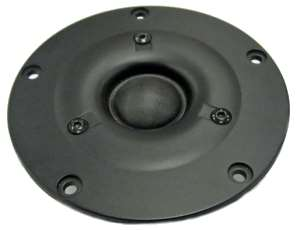GDT-104-N       order no. I-1381639       - not available (_)
25 mm tweeter with high quality cone.
• resonance frequency fs = 1500 Hz
• impedance R = 6 Ohm
• sound pressure level SPL = 92 dB (2,83V; 1m)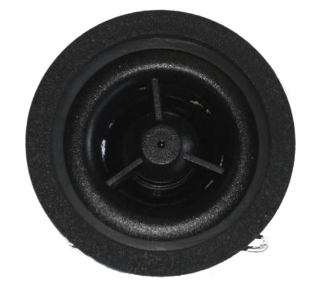open a bigger photo

K10CB       order no. I-1399017       - not available (_)
25 mm tweeter with high quality cone.
• frequency range = 2500-40000 Hz
• impedance R = 8 Ohm
• mounting diameter d = 48 mm
• overall diameter d = 50 mm
• mounting depth (not countersunk) t = 18 mmopen a bigger photo

GRT-175A       order no. I-1381637       EUR 150,00excl. VAT: € 126.05 / \$ 141.18
ribbon tweeter.
• power handling (continuous/programme) = 50/30 W
• frequency range = 2500-40000 Hz
• impedance R = 6 Ohm
• sound pressure level SPL = 96 dB (2,83V; 1m)
• overall diameter d = 175x90 mm
• mass m = 0,65 kg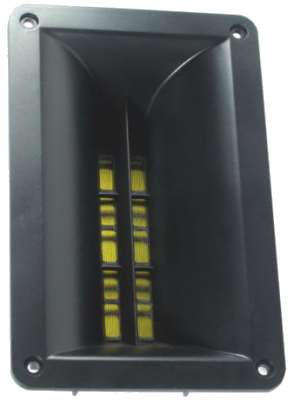GRT-195/MK2       order no. I-1381603- not available
ribbon tweeter.
• power handling (continuous/programme) = 50/85 W
• frequency range = 3000-20000 Hz
• impedance R = 6 Ohm
• sound pressure level SPL = 102 dB (2,83V; 1m)
• overall diameter d = 196x126 mm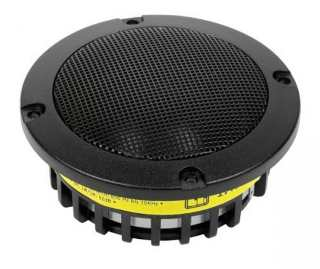open a bigger photo

MD9550       order no. I-5005       - not available (_)
17 cm midrange driver with fabric cone.
• power handling (continuous/programme) = 90/120 W
• frequency range = 750-10000 Hz
• resonance frequency fs = 600 Hz
• impedance R = 4 Ohm
• sound pressure level SPL = 93 dB (2,83V; 1m)
• DC resistance Re = 3,4 Ohm
• voice coil inductance L = 0,077 mH
• total Q factor Qts = 0,64 (Qms=2,75, Qes=0,83)
• voice coil diameter = 50 mm
• mounting diameter d = 78 mm
• overall diameter d = 95 mm
• mounting depth (not countersunk) t = 30 mm

Woofer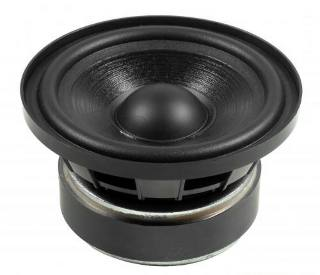open a bigger photo

recommended cabinet 1:
closed cabinet with 0,35 L volume
from 219/138 Hz (-3dB/-8dB)

recommended cabinet 2:
1,2 L volume bass reflex cabinet
with 2cm-Rohr reflex tube, 6 cm long.
from 115/90 Hz (-3dB/-8dB).

TPC80NV       order no. I-1382101       - not available (_)
7 cm full range driver with paper cone.
• power handling (continuous/programme) = 60/80 W
• frequency range = 80-8000 Hz
• resonance frequency fs = 93 Hz
• impedance R = 4 Ohm
• sound pressure level SPL = 83 dB (2,83V; 1m)
• DC resistance Re = 3,3 Ohm
• effective piston radiating area Sd = 35 cm2
• effective mechanical mass incl. air load mms = 2,5 g
• equivalent volume of compliance Vas = 1,9 l
• total Q factor Qts = 0,33 (Qms=2,63, Qes=0,38)
• voice coil diameter = 19 mm
• mounting diameter d = 72 mm
• overall diameter d = 80 mm
• mounting depth (not countersunk) t = 45 mmrecommended cabinet 1:
closed cabinet with 3 L volume
from 111/70 Hz (-3dB/-8dB)

recommended cabinet 2:
12,3 L volume bass reflex cabinet
with HP35 reflex tube, 4 cm long.
from 50/43 Hz (-3dB/-8dB).

GPM 100       order no. I-1381624       - not available (_)
9 cm bass driver with high quality cone.
• power handling (continuous/programme) = 25/50 W
• frequency range = 100-6000 Hz
• resonance frequency fs = 74 Hz
• impedance R = 8 Ohm
• sound pressure level SPL = 84 dB (2,83V; 1m)
• DC resistance Re = 7,36 Ohm
• force factor BL = 3,7 N/A
• effective piston radiating area Sd = 46,6 cm2
• effective mechanical mass incl. air load mms = 3,1 g
• equivalent volume of compliance Vas = 4,5 l
• total Q factor Qts = 0,54 (Qms=1,8, Qes=0,766)
• voice coil diameter = 18 mm
• mounting diameter d = 89 mm
• overall diameter d = 100,5 mm
• mounting depth (not countersunk) t = 53 mm
PDF data sheetGPM 100 TV       order no. I-1381625       - not available (_)
9 cm bass driver with high quality cone. The driver is magnetically shielded.
• power handling (continuous/programme) = 25/50 W
• frequency range = 100-6000 Hz
• resonance frequency fs = 71 Hz
• impedance R = 8 Ohm
• sound pressure level SPL = 84 dB (2,83V; 1m)
• DC resistance Re = 7,4 Ohm
• force factor BL = 3,3 N/A
• effective piston radiating area Sd = 46,6 cm2
• effective mechanical mass incl. air load mms = 3 g
• equivalent volume of compliance Vas = 5 l
• total Q factor Qts = 0,63 (Qms=1,966, Qes=0,927)
• voice coil diameter = 18 mm
• mounting diameter d = 89 mm
• overall diameter d = 100,5 mm
• mounting depth (not countersunk) t = 59,7 mm
PDF data sheetopen a bigger photo

recommended cabinet 1:
closed cabinet with 6 L volume
from 113/71 Hz (-3dB/-8dB)

recommended cabinet 2:
24 L volume bass reflex cabinet
with HP35 reflex tube, 1 cm long.
from 51/44 Hz (-3dB/-8dB).

AX-05       order no. I-1381650       - not available (_)
6 cm full range driver with kevlar cone. The basket is made of die-cast.
• resonance frequency fs = 76,94 Hz
• impedance R = 8 Ohm
• sound pressure level SPL = 90,16 dB (2,83V; 1m)
• DC resistance Re = 6,0 Ohm
• force factor BL = 5,06 N/A
• effective piston radiating area Sd = 85 cm2
• effective mechanical mass incl. air load mms = 5,16 g
• equivalent volume of compliance Vas = 8,35 l
• total Q factor Qts = 0,54 (Qms=7,26, Qes=0,58)
• voice coil diameter = 25 mm
• overall diameter d = 104 mm
PDF data sheetopen a bigger photo

recommended cabinet 1:
closed cabinet with 10 L volume
from 102/65 Hz (-3dB/-8dB)

recommended cabinet 2:
40 L volume bass reflex cabinet
with HP50 reflex tube, 2 cm long.
from 49/40 Hz (-3dB/-8dB).

AX-06       order no. I-1381660       - not available (_)
8 cm full range driver with kevlar cone. The basket is made of die-cast.
• resonance frequency fs = 55,94 Hz
• impedance R = 8 Ohm
• sound pressure level SPL = 92,32 dB (2,83V; 1m)
• DC resistance Re = 5,9 Ohm
• force factor BL = 5,79 N/A
• effective piston radiating area Sd = 137 cm2
• effective mechanical mass incl. air load mms = 7,48 g
• equivalent volume of compliance Vas = 28,29 l
• total Q factor Qts = 0,43 (Qms=7,31, Qes=0,46)
• voice coil diameter = 25 mm
• overall diameter d = 132 mm
PDF data sheet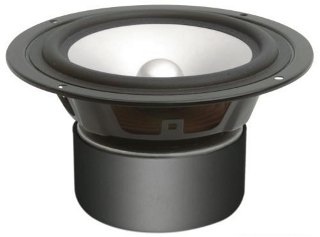open a bigger photo

recommended cabinet 1:
closed cabinet with 2,5 L volume
from 104/65 Hz (-3dB/-8dB)

recommended cabinet 2:
8,1 L volume bass reflex cabinet
with HP35 reflex tube, 9 cm long.
from 55/43 Hz (-3dB/-8dB).

W130AL8       order no. I-1399001       - not available (_)
14 cm full range driver with aluminium cone.
• power handling (continuous/programme) = 75/100 W
• frequency range = 40-6000 Hz
• resonance frequency fs = 44 Hz
• impedance R = 8 Ohm
• sound pressure level SPL = 88 dB (2,83V; 1m)
• DC resistance Re = 6,14 Ohm
• effective piston radiating area Sd = 85 cm2
• effective mechanical mass incl. air load mms = 9,8 g
• equivalent volume of compliance Vas = 13,4 l
• total Q factor Qts = 0,34 (Qms=1,75, Qes=0,42)
• voice coil diameter = 26 mm
• mounting diameter d = 128 mm
• overall diameter d = 147 mm
• mounting depth (not countersunk) t = 79 mm
PDF data sheetopen a bigger photo

MDS05       order no. I-5062       EUR 43,50excl. VAT: € 36.55 / \$ 40.94
14 cm bass driver with kevlar cone. The basket is made of die-cast.
• power handling (continuous/programme) = 100/150 W
• frequency range = -45000 Hz
• resonance frequency fs = 50 Hz
• impedance R = 4 Ohm
• sound pressure level SPL = 88 dB (2,83V; 1m)
• voice coil inductance L = 0,43 mH
• effective piston radiating area Sd = 85 cm2
• effective mechanical mass incl. air load mms = 10,5 g
• equivalent volume of compliance Vas = 9,1 l
• total Q factor Qts = 0,34 (Qms=2,61, Qes=0,39)
• voice coil diameter = 25 mm
• mounting diameter d = 121 mm
• overall diameter d = 150 mm
• mounting depth (not countersunk) t = 77 mm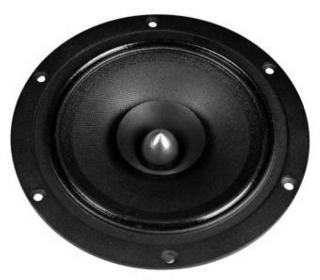open a bigger photo

recommended cabinet 1:
closed cabinet with 54 L volume
from 62/39 Hz (-3dB/-8dB)

recommended cabinet 2:
218 L volume bass reflex cabinet
with HP70 reflex tube, 0 cm long.
from 28/24 Hz (-3dB/-8dB).

AX-08       order no. I-1381680       - not available (_)
11 cm full range driver with kevlar cone. The driver is made with a double voice coil and the given impedance values are for connection in series.
• resonance frequency fs = 41,63 Hz
• impedance R = 8 Ohm
• sound pressure level SPL = 92,19 dB (2,83V; 1m)
• DC resistance Re = 6,3 Ohm
• force factor BL = 5,89 N/A
• effective piston radiating area Sd = 210 cm2
• effective mechanical mass incl. air load mms = 11,67 g
• equivalent volume of compliance Vas = 79,92 l
• total Q factor Qts = 0,53 (Qms=8,91, Qes=0,56)
• voice coil diameter = 25 mm
• overall diameter d = 165 mm
PDF data sheetopen a bigger photo

recommended cabinet 1:
closed cabinet with 9 L volume
from 76/48 Hz (-3dB/-8dB)

recommended cabinet 2:
36 L volume bass reflex cabinet
with HP70 reflex tube, 14 cm long.
from 36/30 Hz (-3dB/-8dB).

W160AL8       order no. I-1399002       - not available (_)
16 cm bass-midrange driver with aluminium cone.
• power handling (continuous/programme) = 80/100 W
• frequency range = 40-4500 Hz
• resonance frequency fs = 44 Hz
• impedance R = 8 Ohm
• sound pressure level SPL = 88 dB (2,83V; 1m)
• DC resistance Re = 6,18 Ohm
• effective piston radiating area Sd = 125 cm2
• effective mechanical mass incl. air load mms = 13 g
• equivalent volume of compliance Vas = 21 l
• total Q factor Qts = 0,46 (Qms=2,13, Qes=0,59)
• voice coil diameter = 26 mm
• mounting diameter d = 143 mm
• overall diameter d = 171 mm
• mounting depth (not countersunk) t = 88 mm
PDF data sheet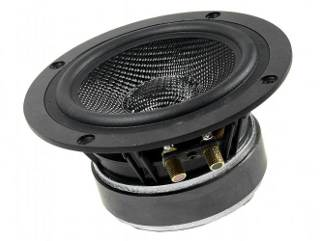open a bigger photo

recommended cabinet 1:
closed cabinet with 7,9 L volume
from 70/44 Hz (-3dB/-8dB)

recommended cabinet 2:
31 L volume bass reflex cabinet
with HP70 reflex tube, 22 cm long.
from 34/27 Hz (-3dB/-8dB).

MDS06       order no. I-5061       EUR 48,00excl. VAT: € 40.34 / \$ 45.18
16 cm bass-midrange driver with kevlar cone. The basket is made of die-cast.
• power handling (continuous/programme) = 100/150 W
• frequency range = -5000 Hz
• resonance frequency fs = 38 Hz
• impedance R = 4 Ohm
• sound pressure level SPL = 88 dB (2,83V; 1m)
• voice coil inductance L = 0,43 mH
• effective piston radiating area Sd = 131 cm2
• effective mechanical mass incl. air load mms = 17,4 g
• equivalent volume of compliance Vas = 22,2 l
• total Q factor Qts = 0,42 (Qms=3,61, Qes=0,48)
• voice coil diameter = 25 mm
• mounting diameter d = 146 mm
• overall diameter d = 181 mm
• mounting depth (not countersunk) t = 84 mm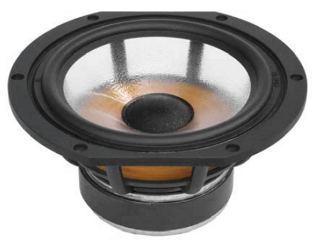open a bigger photo

recommended cabinet 1:
closed cabinet with 6,7 L volume
from 81/51 Hz (-3dB/-8dB)

recommended cabinet 2:
24 L volume bass reflex cabinet
with HP70 reflex tube, 21 cm long.
from 42/33 Hz (-3dB/-8dB).

GDS 182       order no. I-1381630       - not available (_)
17 cm bass-midrange driver with polypropylene cone. The basket is made of die-cast.
• power handling (continuous/programme) = 80/150 W
• frequency range = 40-3000 Hz
• resonance frequency fs = 37,5 Hz
• impedance R = 8 Ohm
• sound pressure level SPL = 87,7 dB (2,83V; 1m)
• DC resistance Re = 5,3 Ohm
• force factor BL = 6,8 N/A
• voice coil inductance L = 0,36 mH
• effective piston radiating area Sd = 137 cm2
• effective mechanical mass incl. air load mms = 15,7 g
• equivalent volume of compliance Vas = 29,9 l
• total Q factor Qts = 0,37 (Qms=2,73, Qes=0,43)
• voice coil diameter = 26 mm
• mounting diameter d = 155 mm
• overall diameter d = 182 mm
• mounting depth (not countersunk) t = 80 mm
• mass m = 1,8 kg
PDF data sheet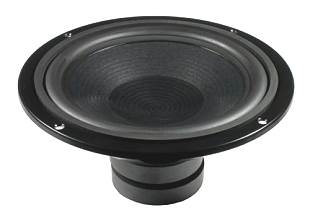GCP 226       order no. I-1381618       - not available (_)
21 cm bass driver with high quality cone.
• power handling (continuous/programme) = 70/140 W
• frequency range = 30-3000 Hz
• impedance R = 8 Ohm
• sound pressure level SPL = 88 dB (2,83V; 1m)
• equivalent volume of compliance Vas = 8,6 l
• voice coil diameter = 25 mm
• mounting diameter d = 186 mm
• overall diameter d = 226 mm
• mounting depth (not countersunk) t = 106 mm
PDF data sheet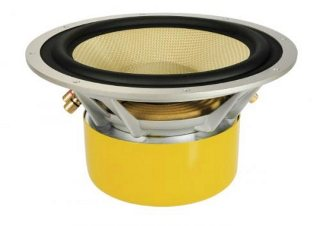open a bigger photo

MDS10DVC       order no. I-1391002       - not available (_)
26 cm bass driver with kevlar cone. The driver is made with a double voice coil and the given impedance values are for connection in series. The speaker has a 4 mm solid metal front plate.
• power handling (continuous/programme) = 300/450 W
• frequency range = -400 Hz
• impedance R = 8 Ohm
• sound pressure level SPL = 90 dB (2,83V; 1m)
• voice coil inductance L = 1,12 mH
• effective piston radiating area Sd = 346 cm2
• effective mechanical mass incl. air load mms = 90,2 g
• equivalent volume of compliance Vas = 47 l
• total Q factor Qts = 0,23 (Qms=7,82, Qes=0,24)
• voice coil diameter = 65 mm
• mounting diameter d = 239 mm
• overall diameter d = 278 mm
• mounting depth (not countersunk) t = 145 mmrecommended cabinet 1:
closed cabinet with 53 L volume
from 52/33 Hz (-3dB/-8dB)

recommended cabinet 2:
199 L volume bass reflex cabinet
with 2x HP100 reflex tube, 21 cm long.
from 26/21 Hz (-3dB/-8dB).

GCP 312/8       order no. I-1381620       - not available (_)
30 cm bass driver with high quality cone.
• power handling (continuous/programme) = 100/300 W
• frequency range = 20-1000 Hz
• resonance frequency fs = 26 Hz
• impedance R = 8 Ohm
• sound pressure level SPL = 90 dB (2,83V; 1m)
• DC resistance Re = 7,16 Ohm
• force factor BL = 11,7 N/A
• effective piston radiating area Sd = 460 cm2
• effective mechanical mass incl. air load mms = 57 g
• equivalent volume of compliance Vas = 194 l
• total Q factor Qts = 0,4 (Qms=2,06, Qes=0,49)
• voice coil diameter = 52 mm
• mounting diameter d = 281 mm
• overall diameter d = 312 mm
• mounting depth (not countersunk) t = 128 mm
PDF data sheetrecommended cabinet 1:
closed cabinet with 39 L volume
from 63/40 Hz (-3dB/-8dB)

recommended cabinet 2:
118 L volume bass reflex cabinet
with 2x HP100 reflex tube, 26 cm long.
from 34/27 Hz (-3dB/-8dB).

GCP 312/4       order no. I-1381619       - not available (_)
30 cm bass driver with high quality cone.
• power handling (continuous/programme) = 100/300 W
• frequency range = 20-1000 Hz
• resonance frequency fs = 24,5 Hz
• impedance R = 4 Ohm
• sound pressure level SPL = 90 dB (2,83V; 1m)
• DC resistance Re = 3,5 Ohm
• force factor BL = 8,3 N/A
• effective piston radiating area Sd = 460 cm2
• effective mechanical mass incl. air load mms = 47,5 g
• equivalent volume of compliance Vas = 263 l
• total Q factor Qts = 0,3 (Qms=1,47, Qes=0,375)
• voice coil diameter = 52 mm
• mounting diameter d = 281 mm
• overall diameter d = 312 mm
• mounting depth (not countersunk) t = 128 mm
PDF data sheetopen a bigger photo

recommended cabinet 1:
closed cabinet with 31 L volume
from 51/32 Hz (-3dB/-8dB)

recommended cabinet 2:
125 L volume bass reflex cabinet
with 2x HP100 reflex tube, 41 cm long.
from 24/20 Hz (-3dB/-8dB).

MDS12DVC       order no. I-5060       EUR 183,60excl. VAT: € 154.29 / \$ 172.80
27 cm bass driver with kevlar cone. The driver is made with a double voice coil and the given impedance values are for connection in series.
• power handling (continuous/programme) = 350/500 W
• frequency range = -250 Hz
• resonance frequency fs = 29,5 Hz
• impedance R = 8 Ohm
• sound pressure level SPL = 89 dB (2,83V; 1m)
• effective piston radiating area Sd = 491 cm2
• effective mechanical mass incl. air load mms = 133 g
• equivalent volume of compliance Vas = 72 l
• total Q factor Qts = 0,46 (Qms=6,56, Qes=0,5)
• voice coil diameter = 65 mm
• mounting diameter d = 179 mm
• overall diameter d = 329 mm
• mounting depth (not countersunk) t = 137 mm

no longer available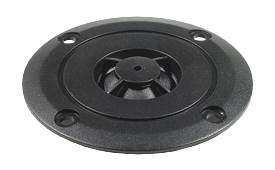GDT 78 neo       order no. I-1381610       - not available (_)
25 mm dome tweeter with fabric dome.
• power handling (continuous/programme) = 20 W
• frequency range = 2500-18000 Hz
• impedance R = 8 Ohm
• sound pressure level SPL = 88 dB (2,83V; 1m)
• voice coil diameter = 25 mm
• mounting diameter d = 54 mm
• overall diameter d = 78 mm
• mounting depth (not countersunk) t = 14,5 mm
PDF data sheet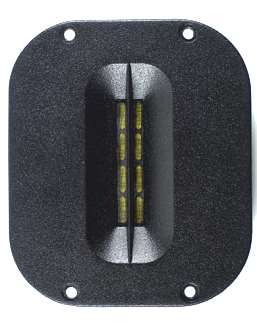GRT 116       order no. I-1381602       - not available (_)
ribbon tweeter. The magnet system is based on Neodymium technology.
• power handling (continuous/programme) = 15 W
• frequency range = 2500-20000 Hz
• impedance R = 6 Ohm
• sound pressure level SPL = 93 dB (2,83V; 1m)
• DC resistance Re = 4,5 Ohm
• voice coil inductance L = - mH
• effective piston radiating area Sd = - cm2
• overall diameter d = 116x85 mm
• mounting depth (not countersunk) t = 33 mm
PDF data sheetGDT 104 R/TV       order no. I-1381622       - not available (_)
25 mm dome tweeter with fabric dome. The driver is magnetically shielded.
• power handling (continuous/programme) = 45 W
• frequency range = 2500-18000 Hz
• impedance R = 8 Ohm
• sound pressure level SPL = 90 dB (2,83V; 1m)
• voice coil diameter = 25 mm
• mounting diameter d = 81 mm
• overall diameter d = 104 mm
• mounting depth (not countersunk) t = 44,8 mm
PDF data sheet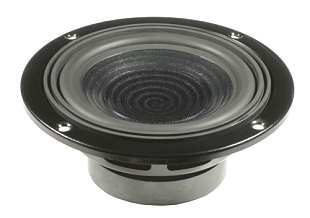recommended cabinet 1:
closed cabinet with 3 L volume
from 93/59 Hz (-3dB/-8dB)

recommended cabinet 2:
11,9 L volume bass reflex cabinet
with HP50 reflex tube, 16 cm long.
from 45/37 Hz (-3dB/-8dB).

GCP 150       order no. I-1381626       - not available (_)
13 cm bass driver with high quality cone.
• power handling (continuous/programme) = 60/120 W
• frequency range = 45-5000 Hz
• resonance frequency fs = 51 Hz
• impedance R = 8 Ohm
• sound pressure level SPL = 84 dB (2,83V; 1m)
• DC resistance Re = 7,65 Ohm
• force factor BL = 6,2 N/A
• effective piston radiating area Sd = 72,3 cm2
• effective mechanical mass incl. air load mms = 8,5 g
• equivalent volume of compliance Vas = 8,5 l
• total Q factor Qts = 0,43 (Qms=2,35, Qes=0,533)
• voice coil diameter = 25 mm
• mounting diameter d = 114 mm
• overall diameter d = 150 mm
• mounting depth (not countersunk) t = 65,5 mm
PDF data sheet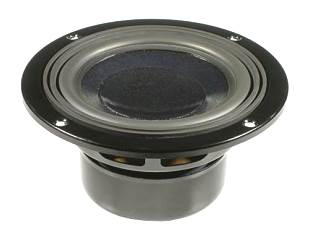recommended cabinet 1:
closed cabinet with 3,3 L volume
from 91/58 Hz (-3dB/-8dB)

recommended cabinet 2:
13 L volume bass reflex cabinet
with HP50 reflex tube, 16 cm long.
from 44/36 Hz (-3dB/-8dB).

GCP 150 TV       order no. I-1381616       - not available (_)
13 cm bass driver with high quality cone. The driver is magnetically shielded.
• power handling (continuous/programme) = 50/100 W
• frequency range = 80-5000 Hz
• resonance frequency fs = 51 Hz
• impedance R = 8 Ohm
• sound pressure level SPL = 84 dB (2,83V; 1m)
• DC resistance Re = 7,8 Ohm
• force factor BL = 6 N/A
• effective piston radiating area Sd = 72,3 cm2
• effective mechanical mass incl. air load mms = 8,4 g
• equivalent volume of compliance Vas = 8,6 l
• total Q factor Qts = 0,45 (Qms=2,12, Qes=0,57)
• voice coil diameter = 26 mm
• mounting diameter d = 114 mm
• overall diameter d = 150 mm
• mounting depth (not countersunk) t = 75 mm
PDF data sheetGCP 188       order no. I-1381617       - not available (_)
17 cm bass driver with high quality cone.
• power handling (continuous/programme) = 60/120 W
• frequency range = 40-4000 Hz
• resonance frequency fs = 43,6 Hz
• impedance R = 8 Ohm
• sound pressure level SPL = 85 dB (2,83V; 1m)
• DC resistance Re = 7,3 Ohm
• force factor BL = 5,76 N/A
• effective piston radiating area Sd = 135 cm2
• effective mechanical mass incl. air load mms = 11,5 g
• equivalent volume of compliance Vas = 29,6 l
• total Q factor Qts = 0,59 (Qms=4,29, Qes=0,69)
• voice coil diameter = 25 mm
• mounting diameter d = 146 mm
• overall diameter d = 188 mm
• mounting depth (not countersunk) t = 80 mm
PDF data sheet
The cabinet volume calculations are executed considering an external resistance of 0.3 Ohm. We are using, if no other data is known or available, the information supplied by the manufacturer.

Please note: the frequency response doesn't say much about the cut-off frequency inside the cabinet! Therefore, we mostly give you 2 examples with dimensioning considerations and an indication, how low the loudspeaker will reproduce.

Home